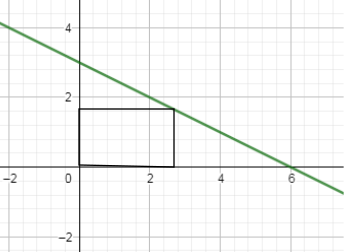# A rectangle is bounded by the x-axis and the y-axis and the graph of \frac{(6-x)}{2}. What length...

## Question:

A rectangle is bounded by the x-axis and the y-axis and the graph of {eq}\frac{(6-x)}{2} {/eq}. What length and width should the rectangle have so that the area is maximum?

## Maximum Area of a Rectangle:

The area of any rectangle is {eq}A=xy {/eq}, where {eq}x {/eq} is the length and {eq}y {/eq} is the width.

If we're given {eq}y {/eq} as a function of {eq}x {/eq}, then we can reduce it to {eq}A=f(x) {/eq} whose maximum value is at {eq}x=c {/eq} when {eq}f'(c)=0 {/eq} and {eq}f''(c) <0 {/eq}.

The process of getting the derivative and then equating the resulting function to {eq}0 {/eq} is accomplished to determine extreme values of any function of only one variable.

A representative rectangle is shown below:The length of the rectangle is {eq}x {/eq} and its width is {eq}y {/eq} so its area is {eq}A=xy {/eq}.

Note that {eq}y=\displaystyle \frac{6-x}{2} {/eq} making {eq}A {/eq} a function of one variable: {eq}\displaystyle A=xy=x\left( \frac{6-x}{2}\right)=-\frac{1}{2}x^2+3x {/eq}.

Setting {eq}A'(x) =0 {/eq}:

{eq}\begin{align*} \displaystyle A'(x)& =0 \\ \frac{\mathrm{d}}{\mathrm{d}x}\left(-\frac{1}{2}x^2+3x\right)& =0 \\ -x+3& =0 \\ x & = 3\\ \end{align*} {/eq}

The second derivative {eq}A''(x)=-1 {/eq} being always negative ensures us that {eq}x=3 {/eq} maximizes {eq}A(x) {/eq}.

If {eq}x=3 {/eq}, {eq}y=\displaystyle \frac{6-x}{2}=\frac{6-3}{2}=\frac{3}{2} {/eq}.

Thus, {eq}3 {/eq} units and {eq}\displaystyle \frac{3}{2} {/eq} units are the length and width, respectively, the rectangle should have so that the area is a maximum.